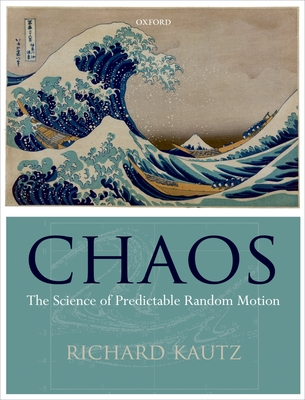### Chaos Chaos (Paperback)

The Science of Predictable Random Motion the Science of Predictable Random Motion

Oxford University Press, USA, 9780199594580, 384pp.

Publication Date: December 30, 2010

List Price: 52.00*
* Individual store prices may vary.

#### Description

Based on only elementary mathematics, this engaging account of chaos theory bridges the gap between introductions for the layman and college-level texts. It develops the science of dynamics in terms of small time steps, describes the phenomenon of chaos through simple examples, and concludes with a close look at a homoclinic tangle, the mathematical monster at the heart of chaos. The presentation is enhanced by many figures, animations of chaotic motion (available on a companion CD), and biographical sketches of the pioneers of dynamics and chaos theory. To ensure accessibility to motivated high school students, care has been taken to explain advanced mathematical concepts simply, including exponentials and logarithms, probability, correlation, frequency analysis, fractals, and transfinite numbers. These tools help to resolve the intriguing paradox of motion that is predictable and yet random, while the final chapter explores the various ways chaos theory has been put to practical use.